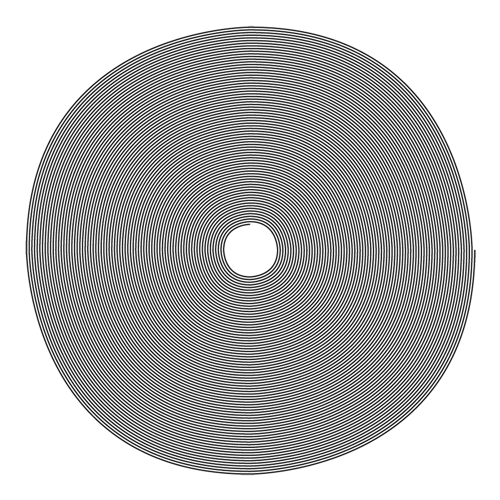# Spiro

extendscript, repeat, form
one single spiro.``````/**
* author: @fabiantheblind
* This script creates a graphic line in circles
* based on this processing sketch by PhiLho
* http://forum.processing.org/topic/how-can-i-draw-this#25080000000995029
* source: https://github.com/ExtendScript/wiki/wiki/Spiro
*/

// the size of the page
var ph = 150;
var pw = 150;

// we need a doc
// use pw and phas size
var doc = app.documents.add({
documentPreferences: {
pageHeight: ph,
pageWidth: pw
}
});

// the page is already there
var page = doc.pages.item(0);
// get the center of the page
var cx = pw / 2;
var cy = ph / 2;
// define the initial radius
var init_rad = 0.9 * (pw / 2);
// this is the amplitude fpr the disturb
var amplitude = radius / 1;

// create a graphicLine
var gl = page.graphicLines.add();
var radians_angle; // this will hold the angle in radians
var rounds = 75; // how many rounds

// ~   we need to count the path points.
// ~   the first an second always exist in  a graphic line
var count = 0;
var degree_step = 4;

// loop through the data.anchors

for(var degrees = 0; degrees < 360 * rounds; degrees += (360 / degree_step)) {

// calculate the degree to radians
radians_angle = ((degrees / 360) % 360) * 2 * Math.PI;
// get the point
var point = gl.paths.pathPoints[count];
// set the disturb to 0 if you want straight lines
// calc the position on the circle
var x1 = cx + (radius) * Math.cos(radians_angle);
var y1 = cy + (radius) * Math.sin(radians_angle);
/**
* a graphicLine always has 2 path points
* so we need to add points only from the third index
*
*/
if(count < 2) {

point.anchor = [x1, y1];

if(count % 4 === 0) {
point.rightDirection = [x1, y1 + radius];
}
if(count % 4 === 1) {
point.rightDirection = [x1 - radius, y1];
}
if(count % 4 === 2) {
point.rightDirection = [x1, y1 - radius];
}
if(count % 4 === 3) {
point.rightDirection = [x1 + radius, y1];
}

}else{
point.anchor = [x1, y1];
if(count % 4 === 0) {
point.rightDirection = [x1, y1 + radius];
}
if(count % 4 === 1) {
point.rightDirection = [x1 - radius, y1];
}
if(count % 4 === 2) {
point.rightDirection = [x1, y1 - radius];
}
if(count % 4 === 3) {
point.rightDirection = [x1 + radius, y1];
}
}

// now make the circle smaller by every round